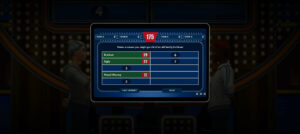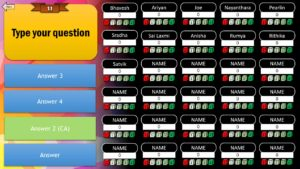This Random PowerPoint Multiplication Flash Cards Generator is created specifically for elementary mathematics teachers and students (3rd to 5th grade).

There is only one slide that updates automatically with new questions. There is also a scoreboard to keep track of the number of questions answered correctly.

It tests the efficiency and accuracy of the student. There is a scoreboard that keeps score of number of multiplication arithmetic questions answered correctly.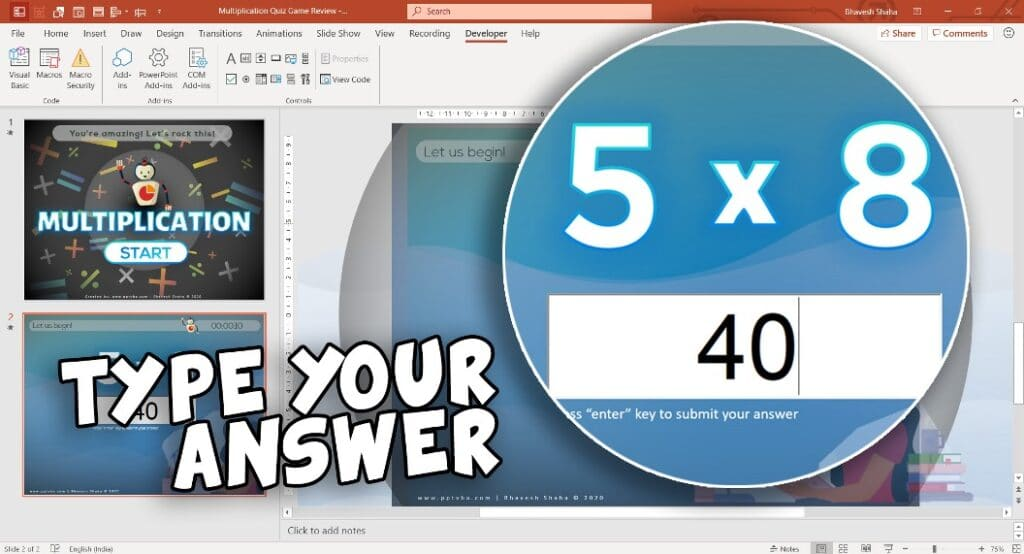These PowerPoint Multiplication Flash Cards are used by 100s of mathematics teachers online classes!

What are you waiting for?

### Generate Two Random Numbers between a given range in PowerPoint VBA

RND function in Visual Basic Application generates a random number of the data type Single which is less than 1 but greater than or equal to 0.

Remember to use the Randomize Function before using the RND Function. The Randomize Function makes the order of the random numbers generated random.

We have to use the following formula in our PowerPoint Maths Games Visual Basic Application code to make sure that the generated random number is between a specific range:

``Q1 = Int((upperbound - lowerbound + 1) * Rnd + lowerbound) ``

Here, upperbound is the highest number in the range, and lowerbound is the lowest number in the range. This allows us to select the range from which the Multiplication Flash Cards would be generated.

We store the generated number within Q1. Similarly, we store another number as Q2.

The two numbers are then shown in the Question Textbox using the .TextFrame.TextRange property of a shape.

``````Sub GenerateQ()

Dim QShp As Shape 'setting shape'
Set QShp = ActivePresentation.Slides(2).Shapes("Q")

'setting range'
L1 = 3
L2 = 15

'generate two random numbers'
Randomize
Q1 = Int((L2 - L1 + 1) * Rnd + L1) 'formula'
Q2 = Int((L2 - L1 + 1) * Rnd + L1) 'formula'

'insert random number in question'
QShp.TextFrame.TextRange = "What is " & Q1 & " x " & Q2

End Sub ``````

### Keep track of Correct & Wrong Multiplication Answers in PowerPoint Maths Games

We use an ActiveX TextBox element which allows the student to type their answer in slide-show mode.

We use an if-then condition to check whether the attempted answer is the same as the correct answer, which is the product of our two integers Q1 & Q2.

Based on that, we increase the number present in our scoreboard: CAShp and WAShp which stands for Correct Answer Shape & WA Answer Shape respectively.

You can use a ActiveX Element Label instead of a Shape to make your work simpler. as the .Caption property would suffice to change the text within the Label.

With the shape, we would have to first define where it exists and then use the .TextFrame.TextRange

``````Sub Initialise()

Set CAShp = ActivePresentation.Slides(2).Shapes("CA")
Set WAShp = ActivePresentation.Slides(2).Shapes("WA")

A.Value = ""
CAShp.TextFrame.TextRange = Int(0)
WAShp.TextFrame.TextRange = Int(0)
End Sub ``````
``````Sub CheckA()

If A.Value = Q1 * Q2 Then
CAShp.TextFrame.TextRange = Int(CAShp.TextFrame.TextRange) + 1
Else
WAShp.TextFrame.TextRange = Int(WAShp.TextFrame.TextRange) + 1
End If

A.Value = ""
GenerateQ

End Sub ``````

After updating the scoreboard, we reset the value of A (Attempted Answer ActiveX TextBox Element) and generate a new Multiplication Question.

### Generate Negative Numbers incase of other operations:

Let us assume our Q1 is equal to a random number that was already generated.
We can introduce a 50-50 probability to convert that into a negative number with the following syntax:

``If Rnd > 0.5 then Q1 = Q1 * (-1) ``

If the answer results in a negative number, the student would have to type the answer following the hyphen symbol – denoting the negative expression,

We can use this multiplication module to create random flashcards for addition & subtraction too. You’d have to just change the range of the random numbers that are generated and you’re good to go!

### Accept only Numbers in TextBox

``````Private Sub A_Change()
If Not IsNumeric(A.Value) Then A.Value = ""
End Sub ``````

### How to generate new question only if the answer is correct

I received a comment on my YouTube video requesting to show the code snippets inorder to create a PowerPoint Multiplication Module in which the new question would be generated only if the question is correct.

``````Sub CheckA()

If A.Value = Q1 * Q2 Then
CAShp.TextFrame.TextRange = Int(CAShp.TextFrame.TextRange) + 1
GenerateQ 'question generated only if correct'
Else
WAShp.TextFrame.TextRange = Int(WAShp.TextFrame.TextRange) + 1
End If

A.Value = ""
End Sub ``````

If you’d want to notify them of the answer incase it is wrong, we can have another shape that would run the following macro to notify them of the answer by inputting it in the ActiveX TextBox on the PowerPoint Game Slide.

``````Sub RevealAnswer()
A.Value = Q1*Q2
End Sub ``````## Who Wants to be a Millionaire? – Download PowerPoint Template Game

Who Wants to be a Millionaire? PowerPoint Template Original Music...These PowerPoint Multiple Choice Quiz Templates perfectly fit the needs...This Customisable Microsoft PowerPoint Fill in the Blanks Template can...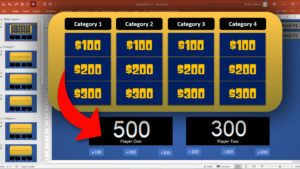## How to CREATE JEOPARDY POWERPOINT GAME WITH SCOREBOARD

Jeopardy PowerPoint Game has an interactive scoreboard for keeping points....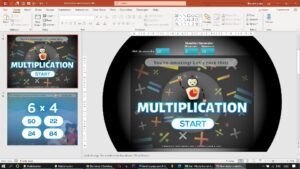## PowerPoint Multiplication Game – Random Flash Card Generator

Only one slide that would generate questions for you automatically....